# Quantum probability and quantum stochastic calculus

### SS 2018

Schedule. Tuesday 16-18, SemR 1.007 and Thursday 10-12, Zeichensaal.

Exam. Week of 23/7-27/7. Second session in September (to be discussed)

### Topics

This course is an introduction to the area of quantum probability and quantum stochastic calculus, seen as non-commutative generalisation of probability theory. This generalisation is attained by replacing the algebra of continuous/measurable functions on a probability space with a C*/von Neumann algebra endowed with a suitable positive linear functional.

Basic understanding of functional analysis and probability theory is required. We will follow mainly the lecture notes of Meyer and Biane which are meant to be understandable by probabilist with no specific training in functional analysis.

For an overview of the main ideas in quantum probability refer to the introductory exposition of Streater:

Streater, R. F. “Classical and Quantum Probability.” Journal of Mathematical Physics 41, no. 6 (May 22, 2000):

3556–3603. https://doi.org/10.1063/1.533322.

### Basic literature

• Meyer, Paul A. Quantum Probability for Probabilists. 2nd edition. Berlin ; New York: Springer, 1995.
• Biane, Philippe. “Calcul Stochastique Non-Commutatif.” In Lectures on Probability Theory, edited by Pierre Bernard, 1–96. Lecture Notes in Mathematics 1608. Springer Berlin Heidelberg, 1995. https://doi.org/10.1007/BFb0095746.

• Parthasarathy, K. R. An Introduction to Quantum Stochastic Calculus. Springer Science & Business Media, 1992.
• Redei, Miklos, and Stephen J. Summers. “Quantum Probability Theory.” ArXiv:Quant-Ph/0601158, January 23, 2006. (arXiv)

### Notes

• Sheet 1 (week 10/4-14/4) (pdf)
• Sheet 2 (week 17/4-19/4) (pdf
• Sheet 3 (week 24/4-26/4) (pdf)
• Sheet 4 (week 8/5) (pdf)
• Sheet 5 (week 15/5-17/5) (pdf)
• Sheet 6 (weeks 29/5 and 5/6-7/6) (pdf)
• Sheet 7 (week 12/6-14/6) (pdf)
• Sheet 8 (week 19/6-21/6) (pdf)
• Sheet 9 (week 3/7-5/7) (pdf)

### Journal

• 10/4 - Introduction, classical and quantum probability. Law of an observable. Quantum Bernoulli space. Pauli matrices.
• 12/4 - Stern-Gerlach experiment, quantum interference. Computation of some basic averages. Pauli matrices as generators of rotations.
• 17/4 - More on impossibility to define the law of non-commuting observables. Heisenberg's uncertainty theorem. Bell's inequalities.
• 19/4 - Coherent states on the Bernoulli space.  More than one Bernoulli variable. Quantum Bernoulli walk. Toy Fock space. Action of creation and annihilation operator.
• 24/4 - Elementary theory of quantum angular momentum. Ladder spaces and representation of the Lie algebra of SU(2). Relation with the quantum Bernoulli walk.
• 26/4 - Representations of rotations and the periodic table of elements. Construction of a commutative Markov process from the magnitude of the quantum Bernoulli walk.
• 1/5 - (no lecture, Tag der Arbeit)
• 3/5 - (cancelled)
• 8/5 -  De Moivre-Laplace theorem for sums of independent quantum Bernoulli variables. The quantum Gaussian variable.
• 10/5 - (no lecture, Himmelfarth)
• 15/5 - Quantum white noise. Construction of the quantum gaussian space (GNS).
• 17/5 - Commutation relations from the pseudo characteristic function. Weyl systems.
• 29/5 - Quantum unbounded random variables: spectral theory of self-adjoint operators, Stone generator of unitary group.
• 31/5 - (no lecture, Froleichnam)
• 5/6 - From a self-adjoint operator back to its spectral measure.
• 7/6 - Structure of a Weyl pair. Stone-von Neumann theorem. Screw lines in Hilbert space.
• 12/6 - End of the proof of the Stone-von Neumann theorem. Schrödinger model of a Weyl pair.
• 14/6 - Alternative proof of the Stone-von Neumann theorem. Reducible actions of a Weyl pair. Some consequences.
• 19/6 - General Gaussian states. Real gaussian model. Creation and annihilation operators. Hermite polynomials.
• 21/6 - Exponential vectors. Complex Gaussian model. Coherent vectors. Non-vacuum states. Computations with the number operator.
• 26/6 -  (cancelled)
• 28/6 -  (cancelled)
• 3/7 - Weyl operators in infinite dimensions. Fock space. Creation and annihilation operators. Exponential vectors and symmetric tensors.
• 5/7 - Second quantisation operators. Some random variables on Fock space. Brownian motion and Poisson process as self-adjoint operators on Fock space.
• 10/7 - (cancelled)
• 12/7 - (cancelled)
• 17/7 -
• 19/7 -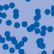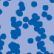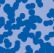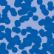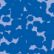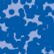## News

Florian Schweiger erhielt den Hausdorff-Gedächtnispreis 2021 der Fachgruppe Mathematik für die beste Dissertation. Er fertigte die Dissertation unter der Betreuung von Prof. Stefan Müller an. Unter anderen wurde Vanessa Ryborz mit einem Preis der Bonner Mathematischen Gesellschaft für ihre von Prof. Sergio Conti betreute Bachelorarbeit ausgezeichnet. (18.01.2022)

Prof. Dr. Sergio Albeverio has been elected into the Academia Europaea and the Accademia Nazionale dei Lincei (more; 02.12.2021).

Der SFB 1060 Die Mathematik der emergenten Effekte hat eine dritte Förderperiode erhalten. (26.11.20)

Prof. Dr. Andreas Eberle erhält den diesjährigen Lehrpreis der Universität Bonn. (22.07.2020)

Herr Dr. Richard Höfer erhielt den Hausdorff-Gedächtnispreis 2019 der Fachgruppe Mathematik für die beste Disseration. Betreut wurde die Arbeit von Prof. J. Velázquez (29.01.2020).

Contact

Managing Director: Prof. Dr. Massimiliano Gubinelli